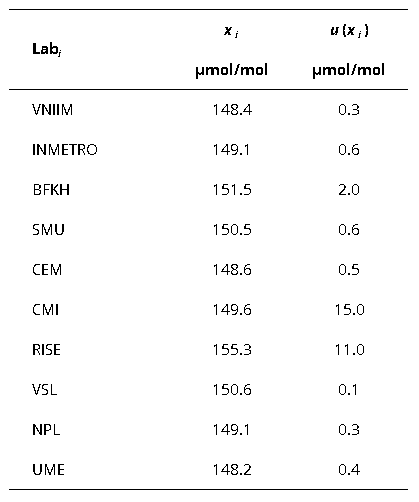Comparison
Comparison close
Results

CCQM-K112

MEASURAND             Contents of biogas / Methane

NOMINAL VALUE     44 cmol/mol

Degrees of equivalence represented by Di = (xI - xR) and its expanded uncertainty Ui at a 95 % level of confidence, both expressed in cmol/mol.

Methane
Di
Participating institutes

CCQM-K112

MEASURAND             Contents of biogas / Carbon dioxide

NOMINAL VALUE     40 cmol/mol

Degrees of equivalence represented by Di = (xI - xR) and its expanded uncertainty Ui at a 95 % level of confidence, both expressed in cmol/mol.

Carbon dioxide
Di
Participating institutes

CCQM-K112

MEASURAND             Contents of biogas / Nitrogen

NOMINAL VALUE     16 cmol/mol

Degrees of equivalence represented by Di = (xI - xR) and its expanded uncertainty Ui at a 95 % level of confidence, both expressed in cmol/mol.

Nitrogen
Di
Participating institutes

CCQM-K112

MEASURAND             Contents of biogas / Hydrogen

NOMINAL VALUE     0.8 cmol/mol

Degrees of equivalence represented by Di = (xI - xR) and its expanded uncertainty Ui at a 95 % level of confidence, both expressed in cmol/mol.

Hydrogen
Di
Participating institutes

CCQM-K112

MEASURAND             Contents of biogas / Oxygen

NOMINAL VALUE     0.5 cmol/mol

Degrees of equivalence represented by Di = (xI - xR) and its expanded uncertainty Ui at a 95 % level of confidence, both expressed in cmol/mol.

Oxygen
Di
Participating institutes

CCQM-K112

MEASURAND             Contents of biogas / Ethane

NOMINAL VALUE     620 µmol/mol

Degrees of equivalence represented by Di = (xI - xR) and its expanded uncertainty Ui at a 95 % level of confidence, both expressed in cmol/mol.

Ethane
Di
Participating institutes

CCQM-K112

MEASURAND             Contents of biogas / Propane

NOMINAL VALUE     150 µmol/mol

Degrees of equivalence represented by Di = (xI - xR) and its expanded uncertainty Ui at a 95 % level of confidence, both expressed in cmol/mol.

Propane
Di
Participating institutes
Comparison
Comparison close
Results

CCQM-K112

MEASURAND             Contents of biogas / Methane

NOMINAL VALUE     44 cmol/mol

Degrees of equivalence represented by Di = (xI - xR) and its expanded uncertainty Ui at a 95 % level of confidence, both expressed in cmol/mol.

LABi Di Uneg,i Upos,i
cmol/mol
cmol/mol
cmol/mol
VNIIM 0.00 0.05
INMETRO 0.04 0.13
BFKH 0.34 0.08
SMU 0.01 0.06
CEM -0.06 0.06
CMI -0.22 0.04
RISE 0.07 0.28
VSL -0.03 0.04
NPL 0.03 0.05
UME 0.01 0.12
Participating institutes
CCQM-K112

CCQM-K112

MEASURAND             Contents of biogas / Carbon dioxide

NOMINAL VALUE     40 cmol/mol

Degrees of equivalence represented by Di = (xI - xR) and its expanded uncertainty Ui at a 95 % level of confidence, both expressed in cmol/mol.

LABi Di Uneg,i Upos,i
cmol/mol
cmol/mol
cmol/mol
VNIIM -0.02 0.06
INMETRO -0.17 0.20
BFKH 0.33 0.05
SMU 0.04 0.12
CEM 0.13 0.16
CMI -0.01 0.03
RISE -0.06 0.25
VSL -0.01 0.04
NPL 0.01 0.04
UME -0.01 0.06
Participating institutes
CCQM-K112

CCQM-K112

MEASURAND             Contents of biogas / Nitrogen

NOMINAL VALUE     16 cmol/mol

Degrees of equivalence represented by Di = (xI - xR) and its expanded uncertainty Ui at a 95 % level of confidence, both expressed in cmol/mol.

LABi Di Uneg,i Upos,i
cmol/mol
cmol/mol
cmol/mol
VNIIM -0.02 0.03
INMETRO 0.12 0.13
BFKH -0.63 0.03
SMU -0.01 0.03
CEM -0.14 0.15
CMI 0.22 0.02
RISE 0.04 0.16
VSL 0.00 0.02
NPL 0.02 0.04
UME 0.01 0.02
Participating institutes
CCQM-K112

CCQM-K112

MEASURAND             Contents of biogas / Hydrogen

NOMINAL VALUE     0.8 cmol/mol

Degrees of equivalence represented by Di = (xI - xR) and its expanded uncertainty Ui at a 95 % level of confidence, both expressed in cmol/mol.

LABi Di Uneg,i Upos,i
cmol/mol
cmol/mol
cmol/mol
VNIIM -0.001 0.004
INMETRO -0.013 0.009
BFKH -0.030 0.012
SMU 0.012 0.013
CEM -0.012 0.232
CMI -0.004 0.061
RISE 0.014 0.017
VSL -0.001 0.003
NPL 0.004 0.004
UME 0.001 0.003
Participating institutes
CCQM-K112

CCQM-K112

MEASURAND             Contents of biogas / Oxygen

NOMINAL VALUE     0.5 cmol/mol

Degrees of equivalence represented by Di = (xI - xR) and its expanded uncertainty Ui at a 95 % level of confidence, both expressed in cmol/mol.

LABi Di Uneg,i Upos,i
cmol/mol
cmol/mol
cmol/mol
VNIIM 0.000 0.002
INMETRO -0.002 0.003
BFKH -0.018 0.002
SMU 0.001 0.004
CEM 0.024 0.003
CMI 0.002 0.011
RISE -0.011 0.011
VSL 0.003 0.003
NPL 0.002 0.002
UME -0.019 0.002
Participating institutes
CCQM-K112

CCQM-K112

MEASURAND             Contents of biogas / Ethane

NOMINAL VALUE     620 µmol/mol

Degrees of equivalence represented by Di = (xI - xR) and its expanded uncertainty Ui at a 95 % level of confidence, both expressed in cmol/mol.

LABi Di Uneg,i Upos,i
µmol/mol
µmol/mol
µmol/mol
VNIIM -1.4 1.9
INMETRO 0.1 2.4
BFKH 1.5 7.7
SMU -0.3 4.9
CEM -0.1 1.5
CMI -12.4 58.6
RISE 48.2 34.4
VSL 1.1 1.6
NPL -0.6 2.5
UME -0.9 3.3
Participating institutes
CCQM-K112

CCQM-K112

MEASURAND             Contents of biogas / Propane

NOMINAL VALUE     150 µmol/mol

Degrees of equivalence represented by Di = (xI - xR) and its expanded uncertainty Ui at a 95 % level of confidence, both expressed in cmol/mol.

LABi Di Uneg,i Upos,i
µmol/mol
µmol/mol
µmol/mol
VNIIM -0.9 1.0
INMETRO -0.2 1.3
BFKH 2.1 3.8
SMU 1.2 1.3
CEM -0.7 1.2
CMI 0.4 29.1
RISE 6.0 21.3
VSL 1.3 0.8
NPL -0.2 0.9
UME -1.1 1.1
Participating institutes
CCQM-K112
Comparison
Comparison close
CC comparison
 Metrology area, Sub-field Chemistry and Biology, Gases Description Composition of Biogas Time of measurements 2014 Status Approved for equivalence
 Final Reports of the comparisons Transfer device Dedicated samples
 Comparison type Key Comparison Consultative Committee CCQM (Consultative Committee for Amount of Substance) Conducted by CCQM (Consultative Committee for Amount of Substance) Comments Results published on 4 August 2020 Pilot institute VSL VSL Netherlands Contact person A. van der Veen avdveen@vsl.nl +31 15 2691500
First Name Last Name
wwww@ww.www +356719836 Institute 1 Institute 1 Khmelnitskiy
Pilot laboratory
VSL

VSL, Netherlands, EURAMET

 BFKH Government Office of the Capital City Budapest, Hungary, EURAMET
 CEM Centro Español de Metrologia, Spain, EURAMET
 CMI Czech Metrology Institute, Czechia, EURAMET
 INMETRO Instituto Nacional de Metrologia, Qualidade e Tecnologia, Brazil, SIM
 NPL National Physical Laboratory, United Kingdom, EURAMET
 RISE Research Institutes of Sweden AB, Sweden, EURAMET
 SMU Slovensky Metrologicky Ustav, Slovakia, EURAMET
 UME TÜBITAK Ulusal Metroloji Enstitüsü, Turkey, EURAMET
 VNIIM D.I. Mendeleyev Institute for Metrology, Rosstandart, Russian Federation, COOMET
Comparison
Comparison close
Results

CCQM-K112

MEASURAND             Contents of biogas / Methane

NOMINAL VALUE     44 cmol/mol

The key comparison reference value xR and its associated standard uncertainty (xR) are consensus values for each component, computed using two different methods. For more information, cf. CCQM-K112 Final Report (Sec. 3.4).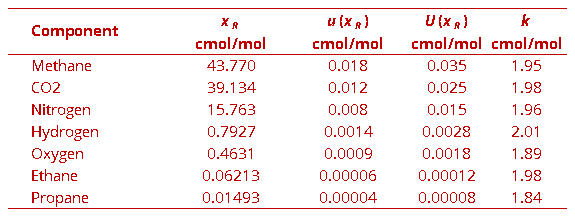The degree of equivalence of laboratory i relative to the key comparison reference value xR is given by a pair of terms:

Di = (xi - xR) and its expanded uncertainty Ui at a 95 % level of confidence where Uik u, where k represents the coverage factor.

CCQM-K112

MEASURAND             Contents of biogas / Carbon diaoxide

NOMINAL VALUE     40 cmol/mol

The key comparison reference value xR and its associated standard uncertainty (xR) are consensus values for each component, computed using two different methods. For more information, cf. CCQM-K112 Final Report (Sec. 3.4).The degree of equivalence of laboratory i relative to the key comparison reference value xR is given by a pair of terms:

Di = (xi - xR) and its expanded uncertainty Ui at a 95 % level of confidence where Uik u, where k represents the coverage factor.

CCQM-K112

MEASURAND             Contents of biogas / Nitrogen

NOMINAL VALUE     16 cmol/mol

The key comparison reference value xR and its associated standard uncertainty (xR) are consensus values for each component, computed using two different methods. For more information, cf. CCQM-K112 Final Report (Sec. 3.4).The degree of equivalence of laboratory i relative to the key comparison reference value xR is given by a pair of terms:

Di = (xi - xR) and its expanded uncertainty Ui at a 95 % level of confidence where Uik u, where k represents the coverage factor.

CCQM-K112

MEASURAND             Contents of biogas / Hydrogen

NOMINAL VALUE     0.8 cmol/mol

The key comparison reference value xR and its associated standard uncertainty (xR) are consensus values for each component, computed using two different methods. For more information, cf. CCQM-K112 Final Report (Sec. 3.4).The degree of equivalence of laboratory i relative to the key comparison reference value xR is given by a pair of terms:

Di = (xi - xR) and its expanded uncertainty Ui at a 95 % level of confidence where Uik u, where k represents the coverage factor.

CCQM-K112

MEASURAND             Contents of biogas / Oxygen

NOMINAL VALUE     0.5 cmol/mol

The key comparison reference value xR and its associated standard uncertainty (xR) are consensus values for each component, computed using two different methods. For more information, cf. CCQM-K112 Final Report (Sec. 3.4).The degree of equivalence of laboratory i relative to the key comparison reference value xR is given by a pair of terms:

Di = (xi - xR) and its expanded uncertainty Ui at a 95 % level of confidence where Uik u, where k represents the coverage factor.

CCQM-K112

MEASURAND             Contents of biogas / Ethane

NOMINAL VALUE     620 µmol/mol

The key comparison reference value xR and its associated standard uncertainty (xR) are consensus values for each component, computed using two different methods. For more information, cf. CCQM-K112 Final Report (Sec. 3.4).The degree of equivalence of laboratory i relative to the key comparison reference value xR is given by a pair of terms:

Di = (xi - xR) and its expanded uncertainty Ui at a 95 % level of confidence where Uik u, where k represents the coverage factor.

CCQM-K112

MEASURAND             Contents of biogas / Propane

NOMINAL VALUE     150 µmol/mol

The key comparison reference value xR and its associated standard uncertainty (xR) are consensus values for each component, computed using two different methods. For more information, cf. CCQM-K112 Final Report (Sec. 3.4).The degree of equivalence of laboratory i relative to the key comparison reference value xR is given by a pair of terms:

Di = (xi - xR) and its expanded uncertainty Ui at a 95 % level of confidence where Uik u, where k represents the coverage factor.

Comparison
Comparison close
Results

CCQM-K112

MEASURAND             Contents of biogas / Methane

NOMINAL VALUE     44 cmol/mol

xi   measured amount fraction reported by laboratory iLabi

ui   combined standard uncertainty of xi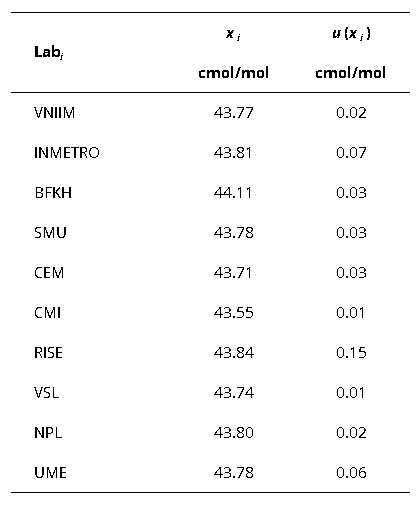CCQM-K112

MEASURAND             Contents of biogas / Carbon dioxide

NOMINAL VALUE     40 cmol/mol

xi   measured amount fraction reported by laboratory iLabi

ui   combined standard uncertainty of xi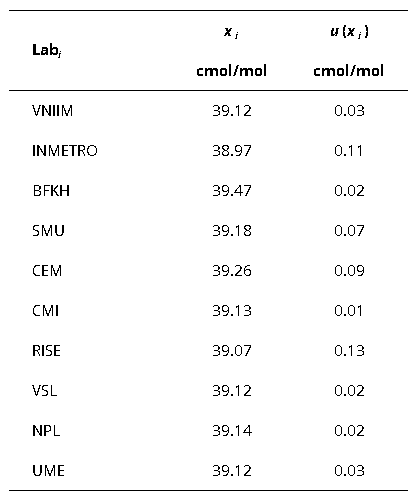CCQM-K112

MEASURAND             Contents of biogas / Nitrogen

NOMINAL VALUE     16 cmol/mol

xi   measured amount fraction reported by laboratory iLabi

ui   combined standard uncertainty of xi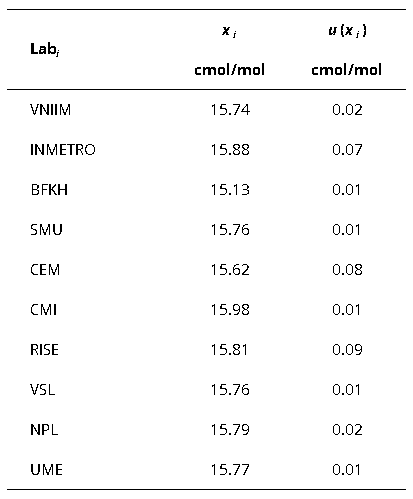CCQM-K112

MEASURAND             Contents of biogas / Hydrogen

NOMINAL VALUE     0.8 cmol/mol

xi   measured amount fraction reported by laboratory iLabi

ui   combined standard uncertainty of xi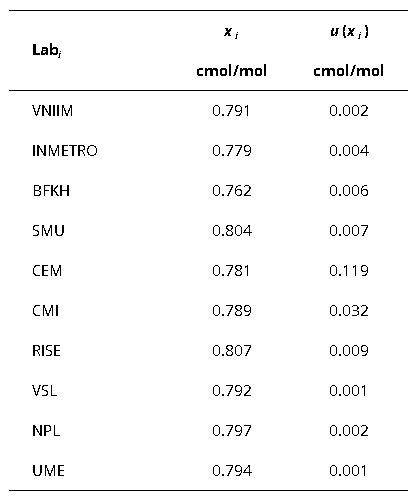CCQM-K112

MEASURAND             Contents of biogas / Oxygen

NOMINAL VALUE     0.5 cmol/mol

xi   measured amount fraction reported by laboratory iLabi

ui   combined standard uncertainty of xi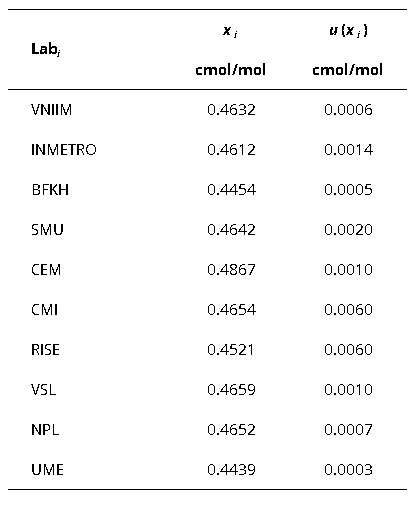CCQM-K112

MEASURAND             Contents of biogas / Ethane

NOMINAL VALUE     620 µmol/mol

xi   measured amount fraction reported by laboratory iLabi

ui   combined standard uncertainty of xi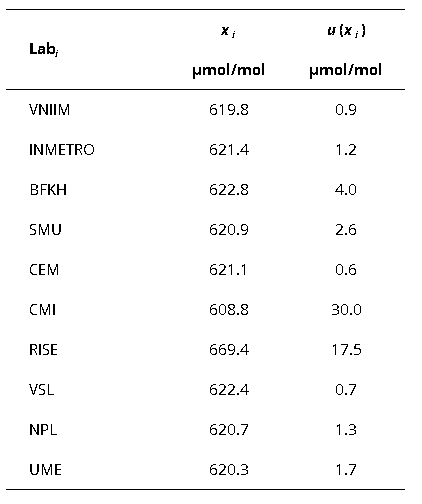CCQM-K112

MEASURAND             Contents of biogas / Propane

NOMINAL VALUE     150 µmol/mol

xi   measured amount fraction reported by laboratory iLabi

ui   combined standard uncertainty of xi